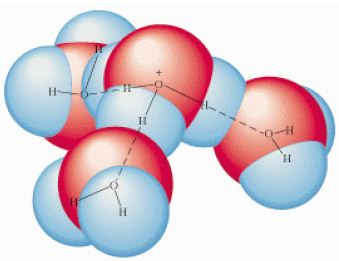Chemistry

# Arrhenius Theory

According to the Arrhenius theory an acid is a substance that, when dissolved in water, produces hydronium ions (H3O+) as follows:

HCl (I) + H2O (I) → H3O+ (aq) + Cl (aq)

Chemists often simply call this the hydrogen ion, and use the notation H+ (aq) as shorthand for the H3O+ (aq) ion.

Be aware, however, that the hydrogen ion H+ in solution is actually chemically bonded to water, i.e. H3O+.Hydrogen bonding makes the hydrated ion even larger: the H3O+ shown above forms hydrogen bonding with three more water molecules and therefore is usually just written H+ (aq).

Similarly, the Arrhenius theory states that when bases dissolve in water, they form hydroxide ions, OH as shown below:

Na2O + H2O → 2 Na+ + 2 OH

The Arrhenius theory is limited in that it looks at acids and bases in aqueous solutions only. In addition, it singles out the OH ion as the source of the base character, when other species can play a similar role. Broader definitions of acids and bases were then developed.